# Andhra Pradesh SSC Board Question Paper for Class 10th Maths Paper 2 2018 In PDF

## AP SSC or 10th Class Question Paper Mathematics Paper 2 English Medium 2018 with Solutions – Free Download

Andhra Pradesh SSC (Class 10) Maths 2018 question paper 2 with solutions are provided here in a downloadable pdf format and also in the text so that the students can easily get them. Along with the solutions, they can also download the Maths question paper 2 2018 class 10 SSC for reference. Students are open to access all the Andhra Pradesh board previous year Maths question papers here. 10th Class Mathematics Question Paper 2018 Paper 2 can be downloaded easily and students can practice and verify the answers provided by BYJU’S. Solving 2018 Maths question paper 2 for Class 10 will help the students to predict what type of questions will appear in the exam.

### QUESTION PAPER CODE 16E(A)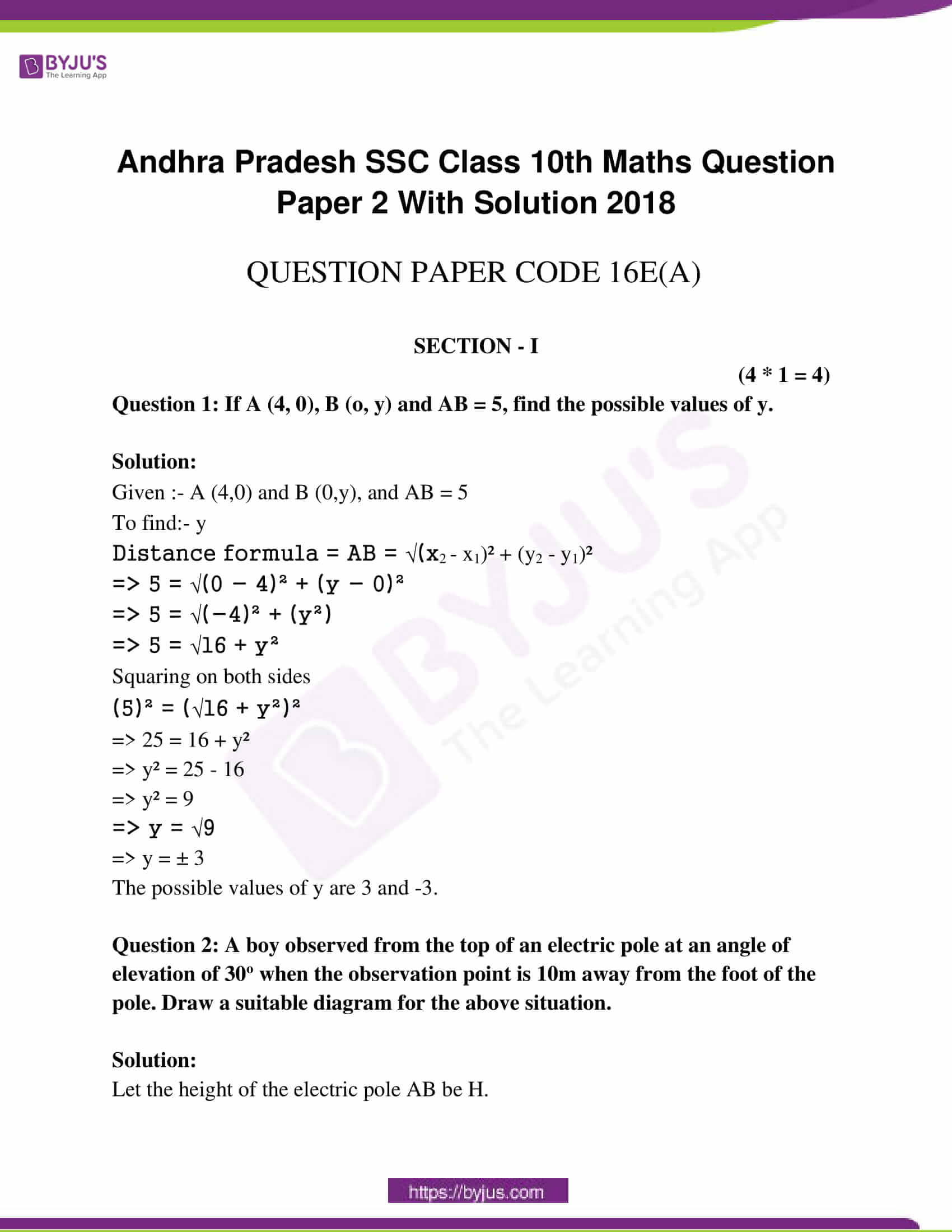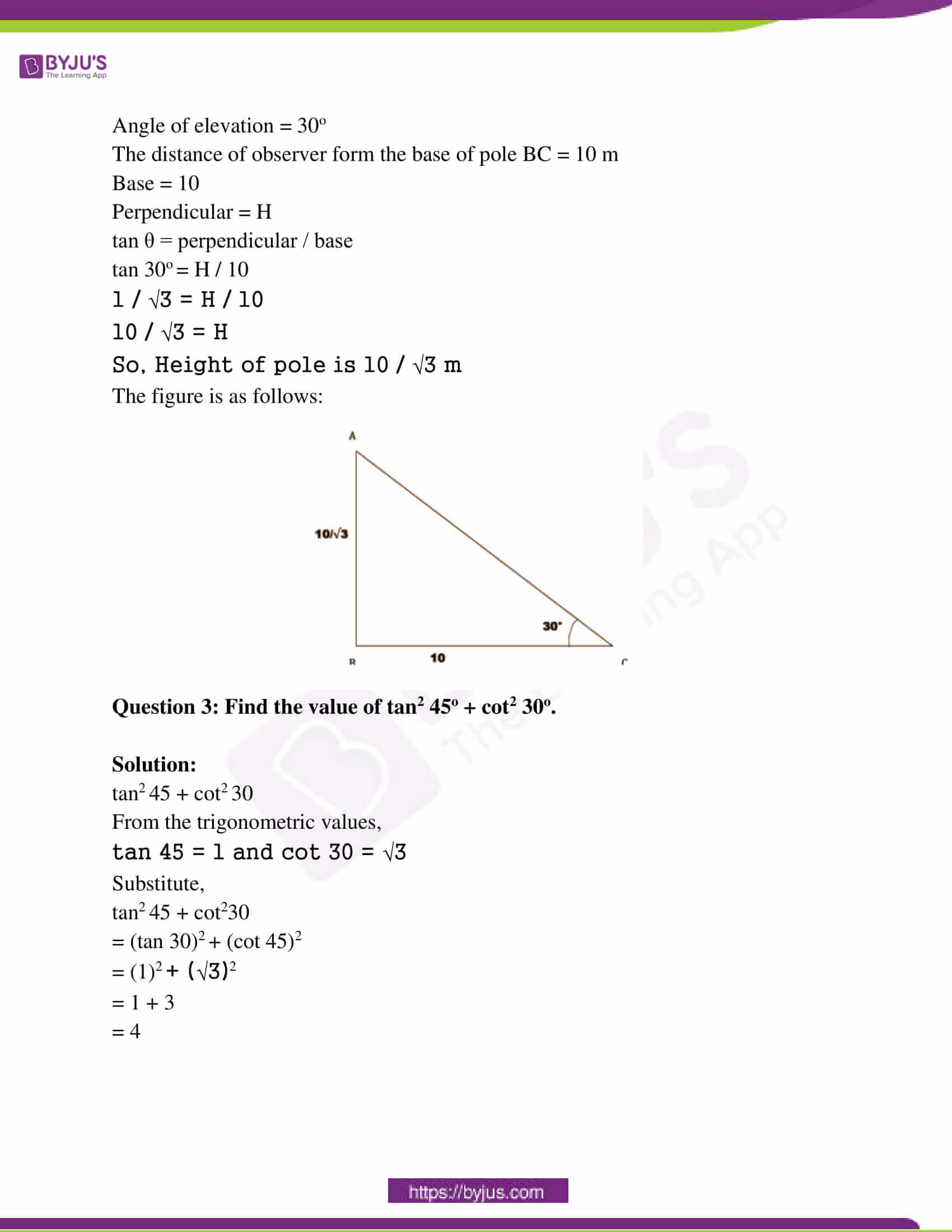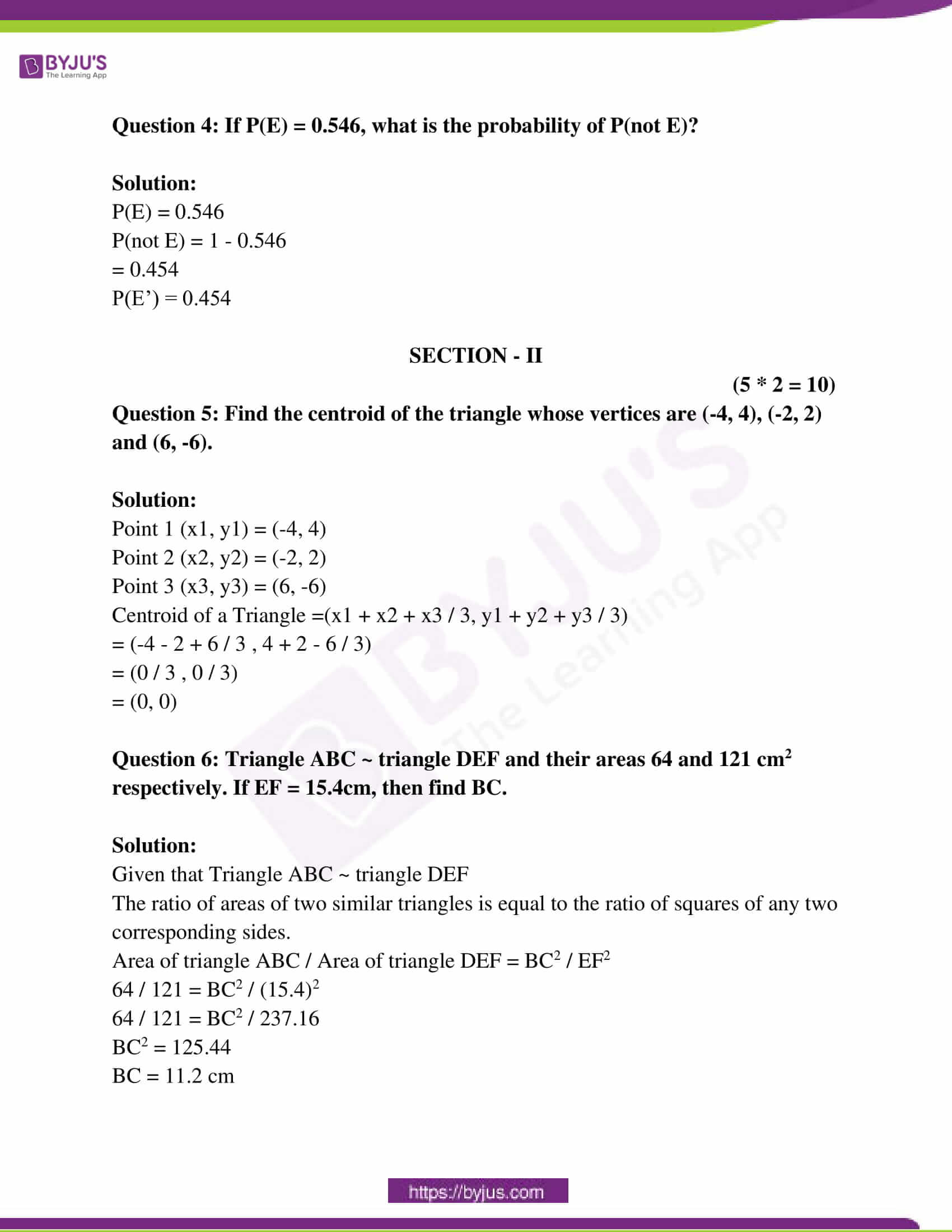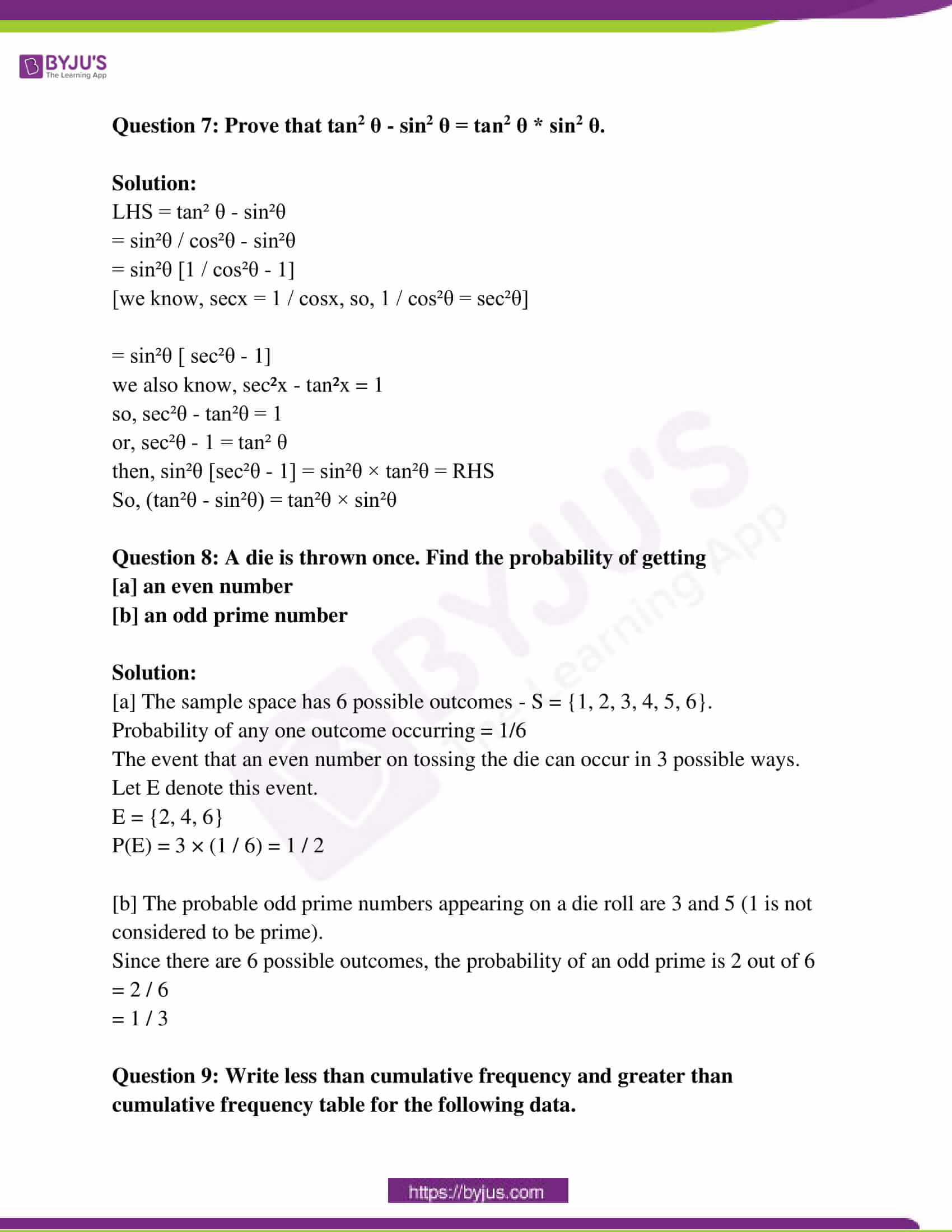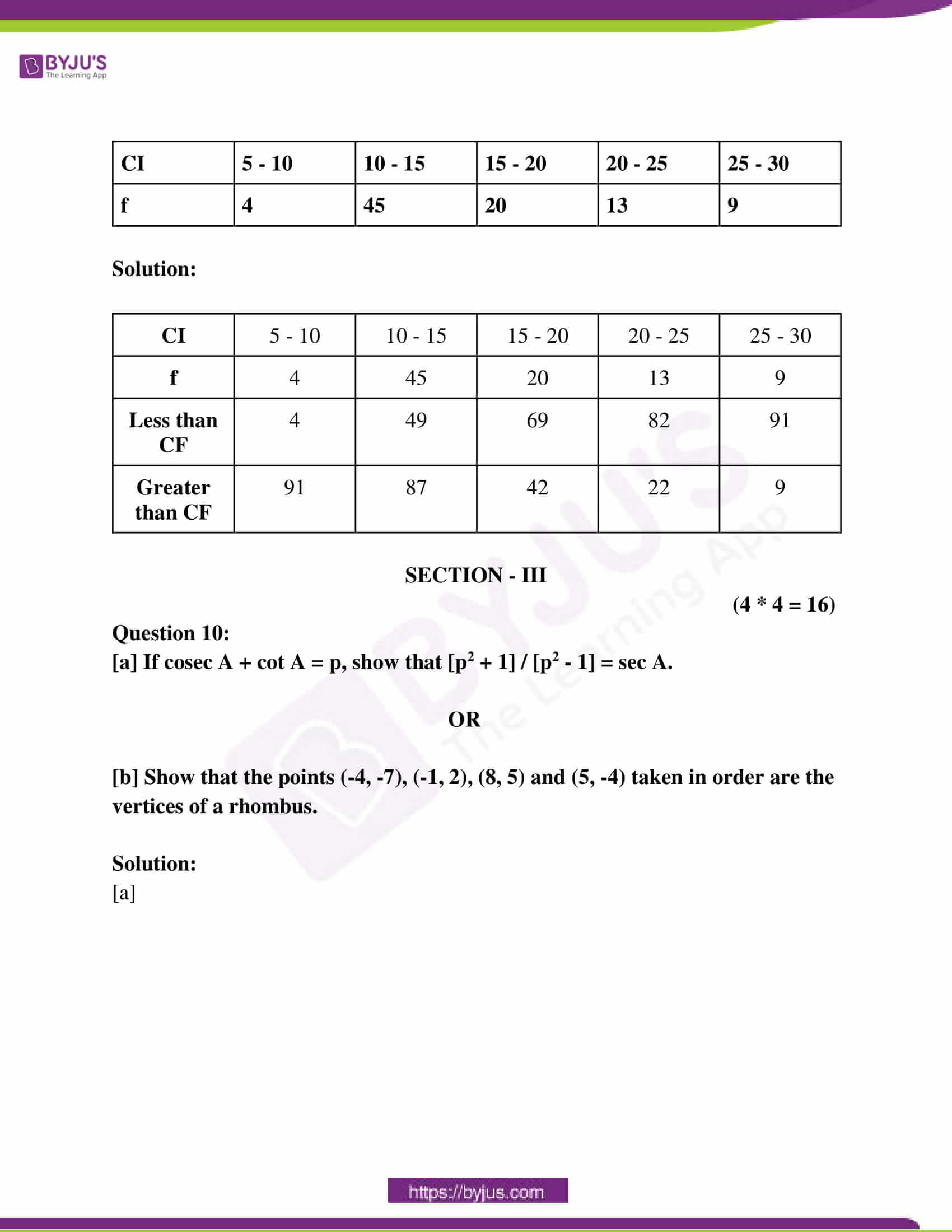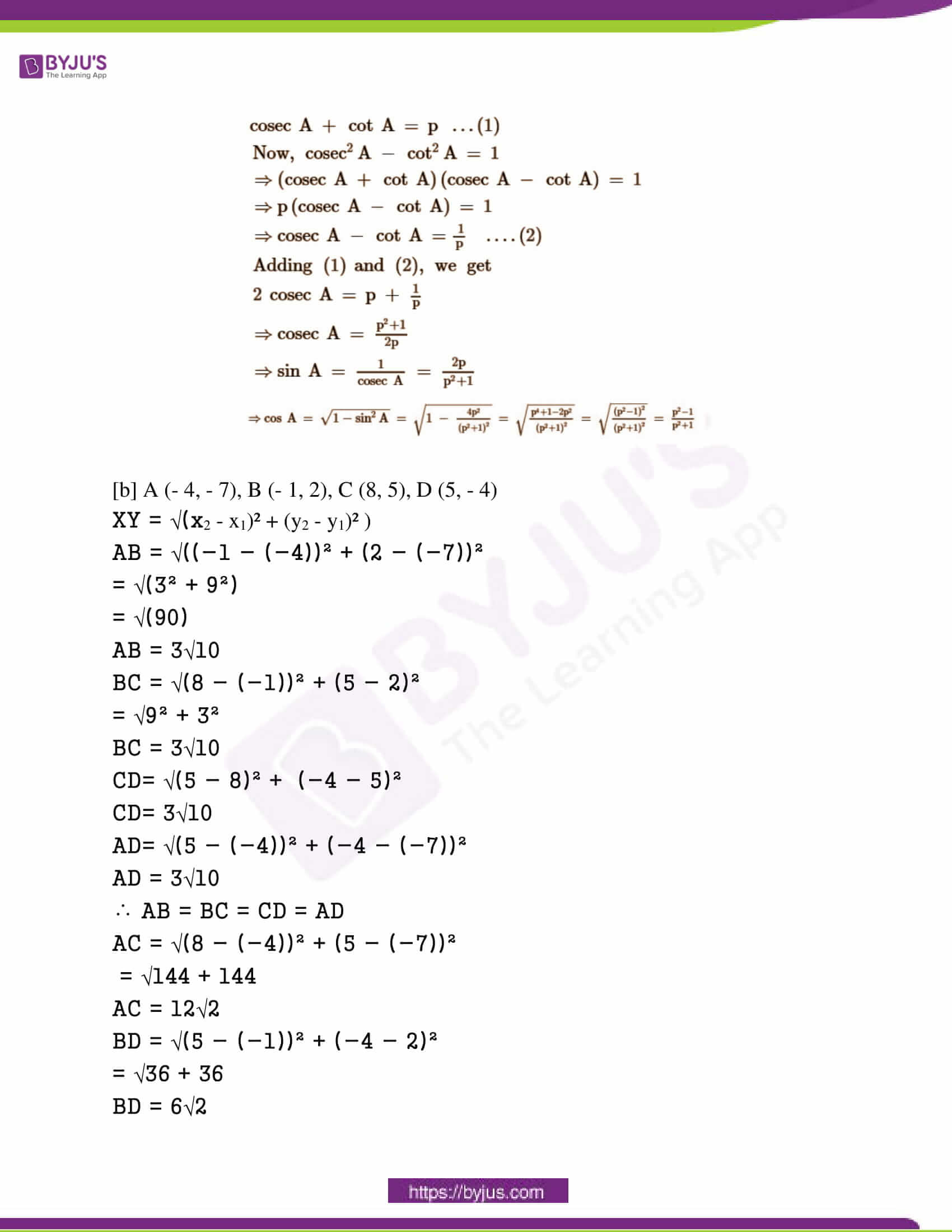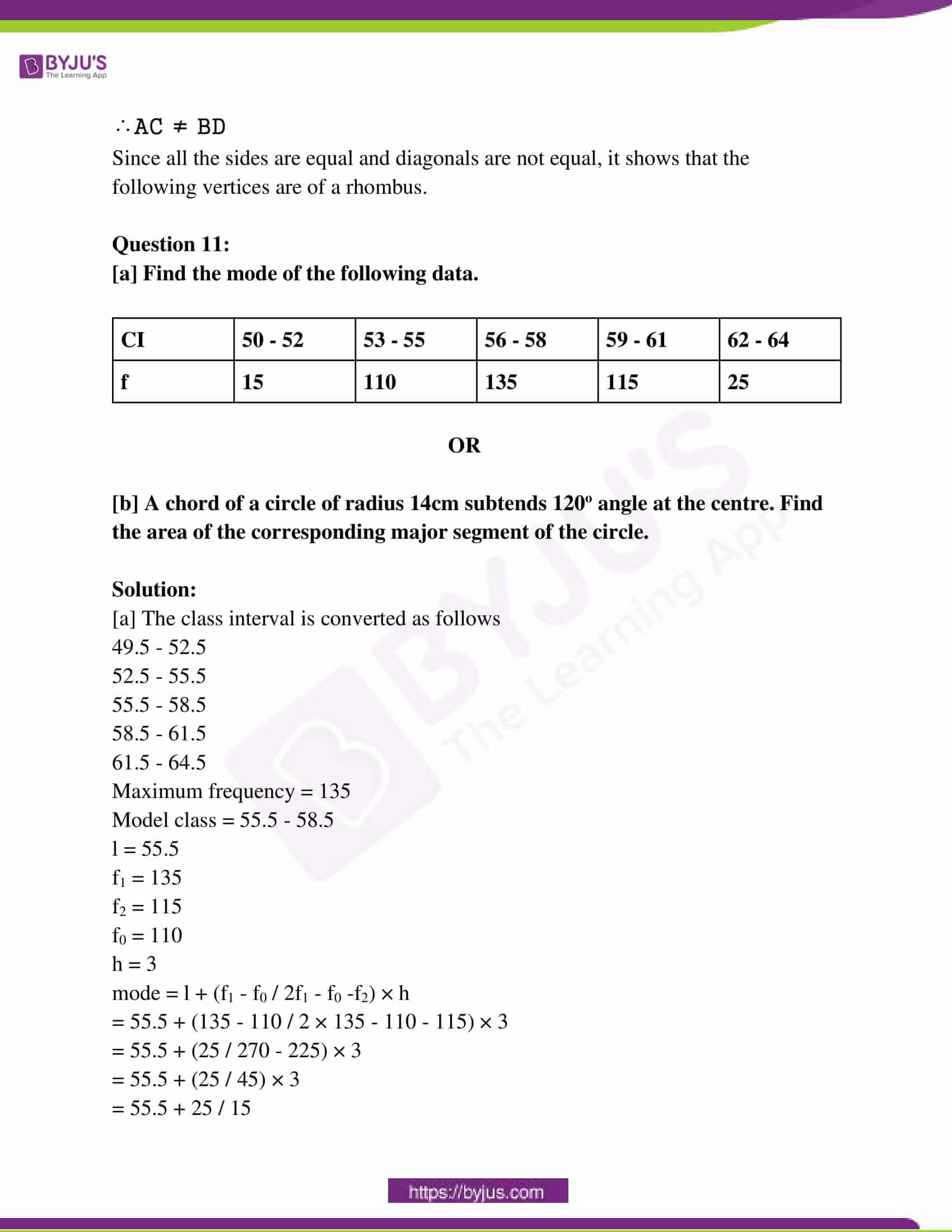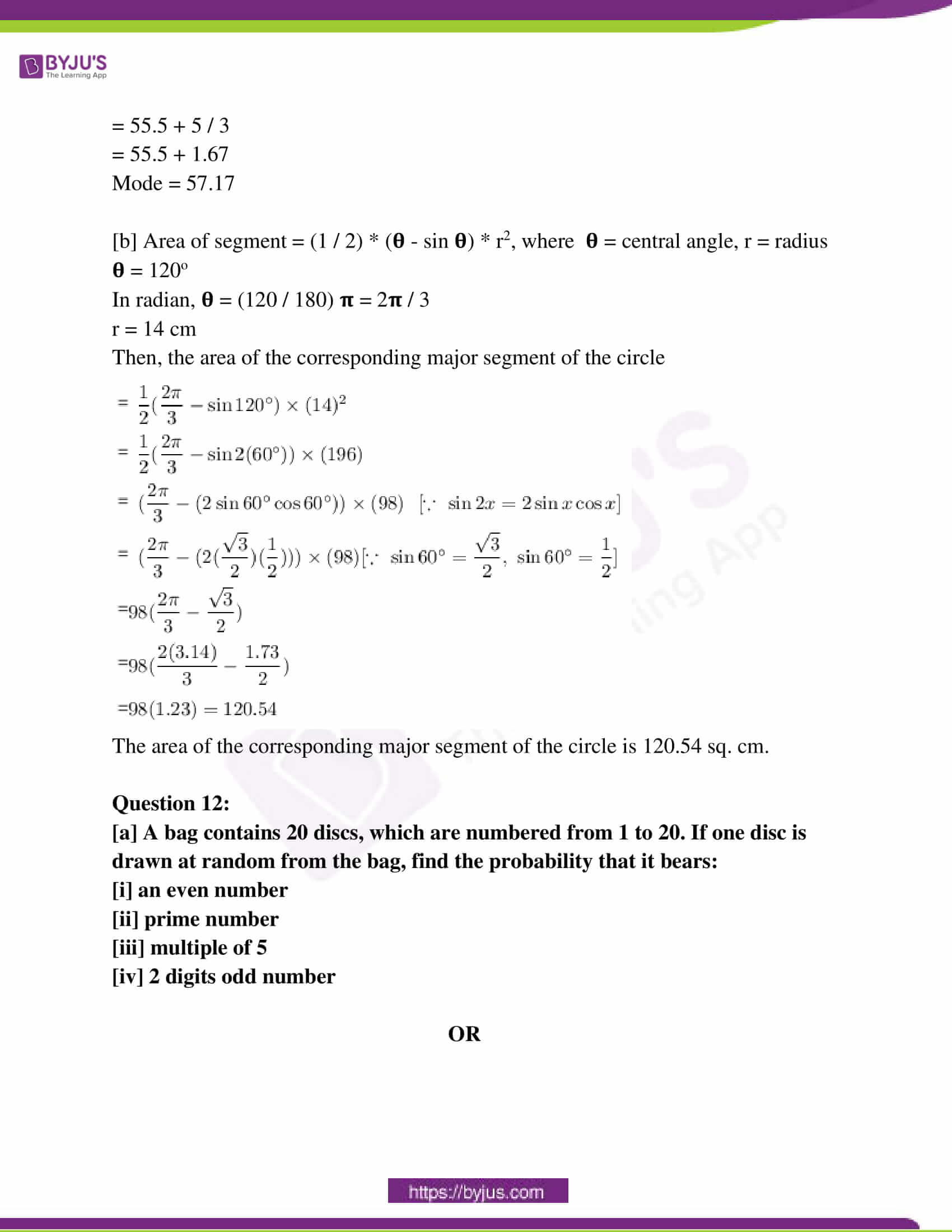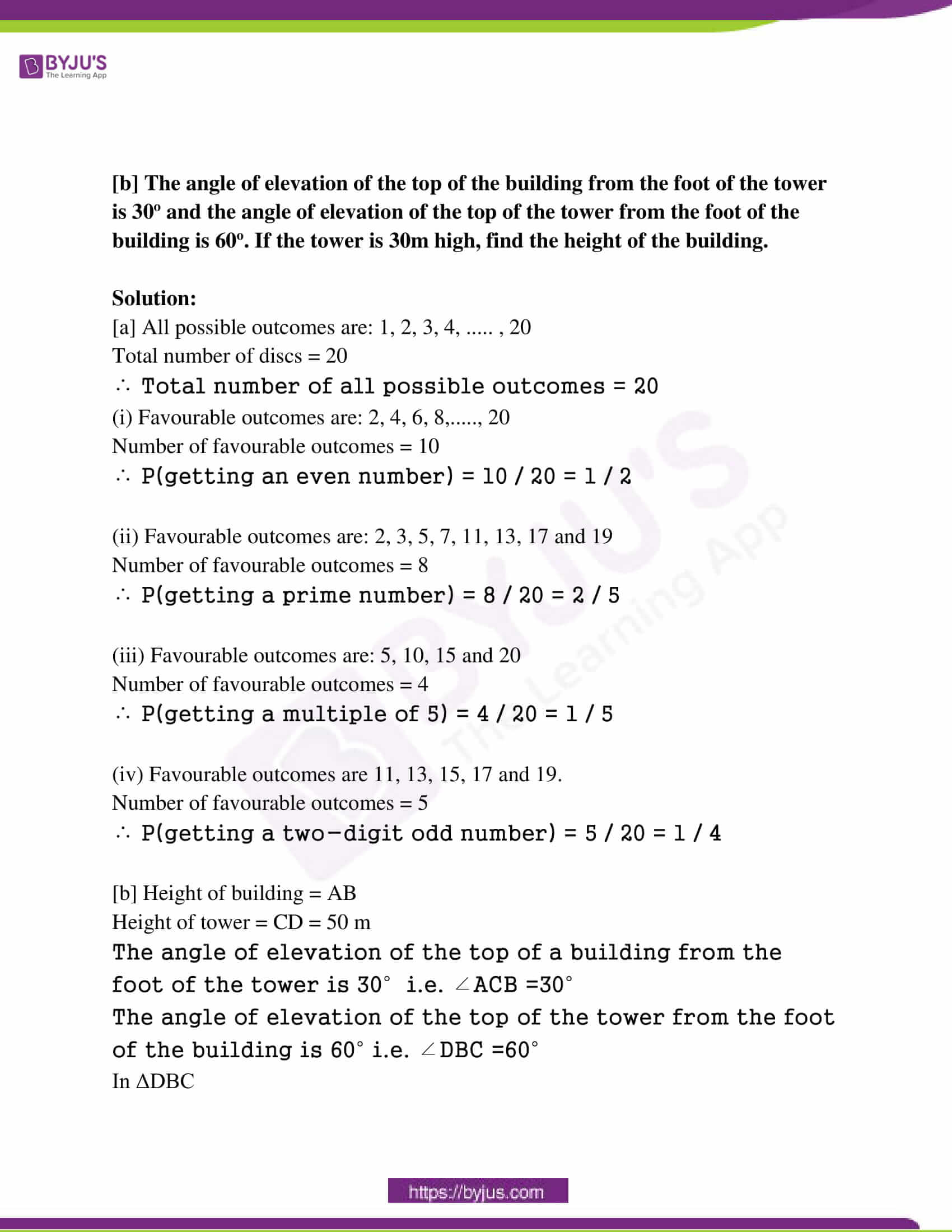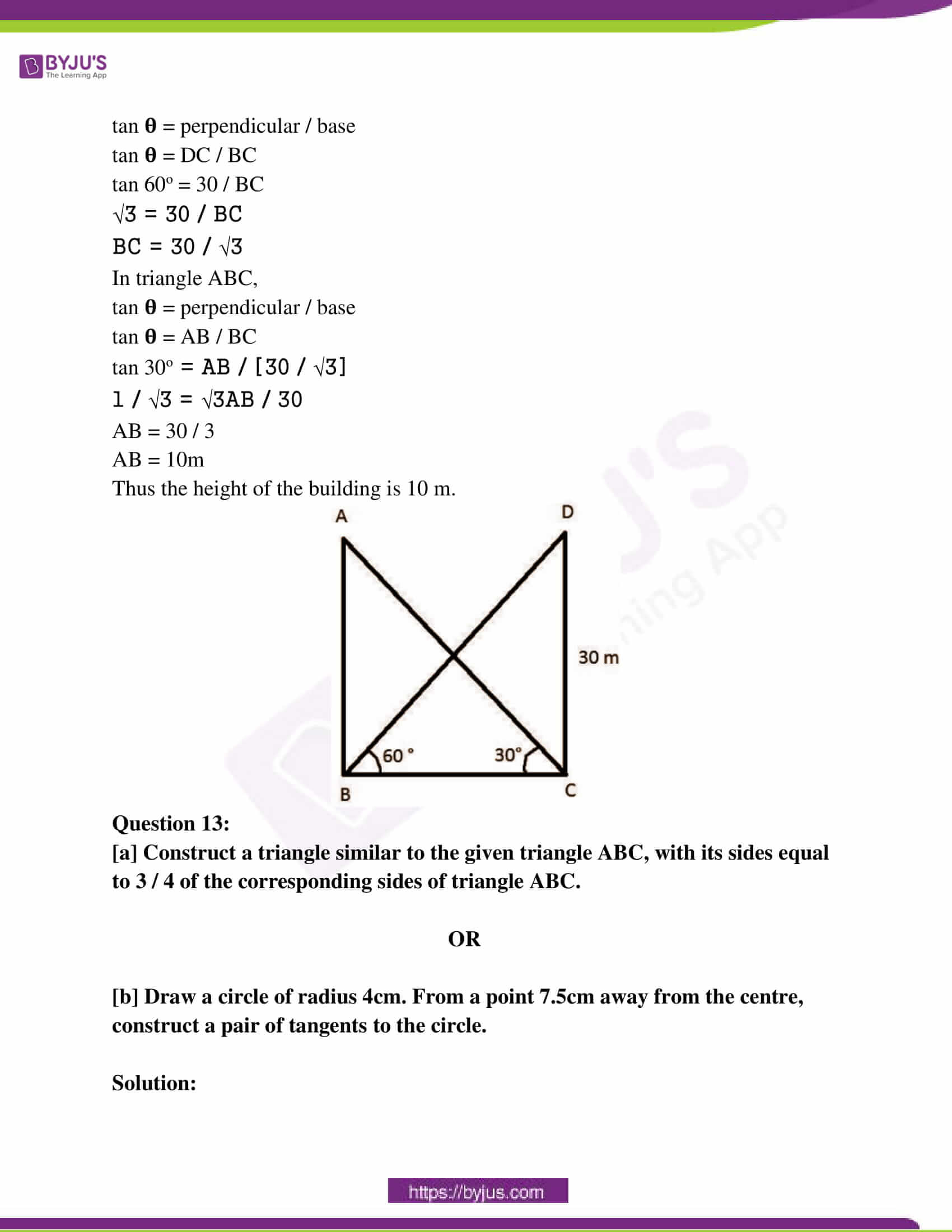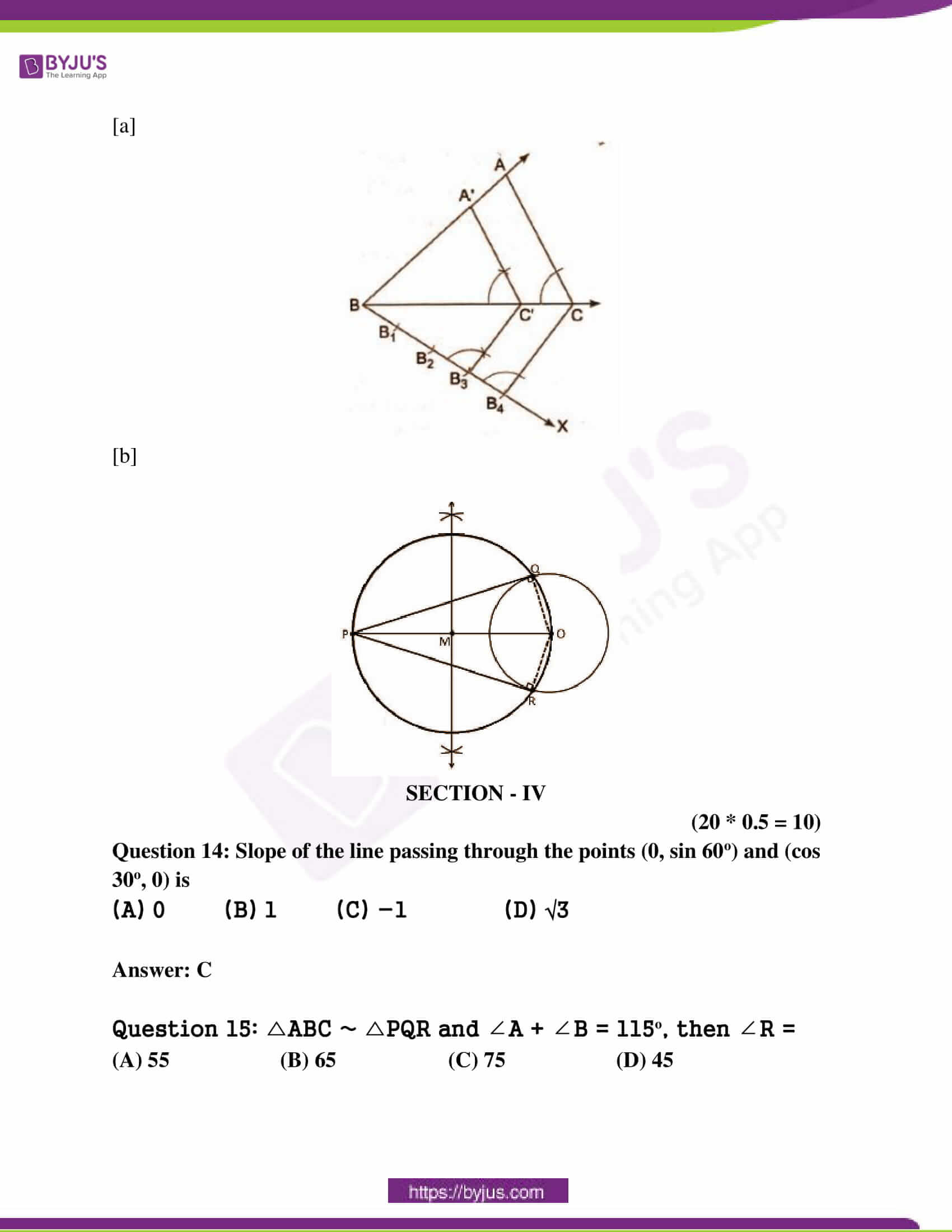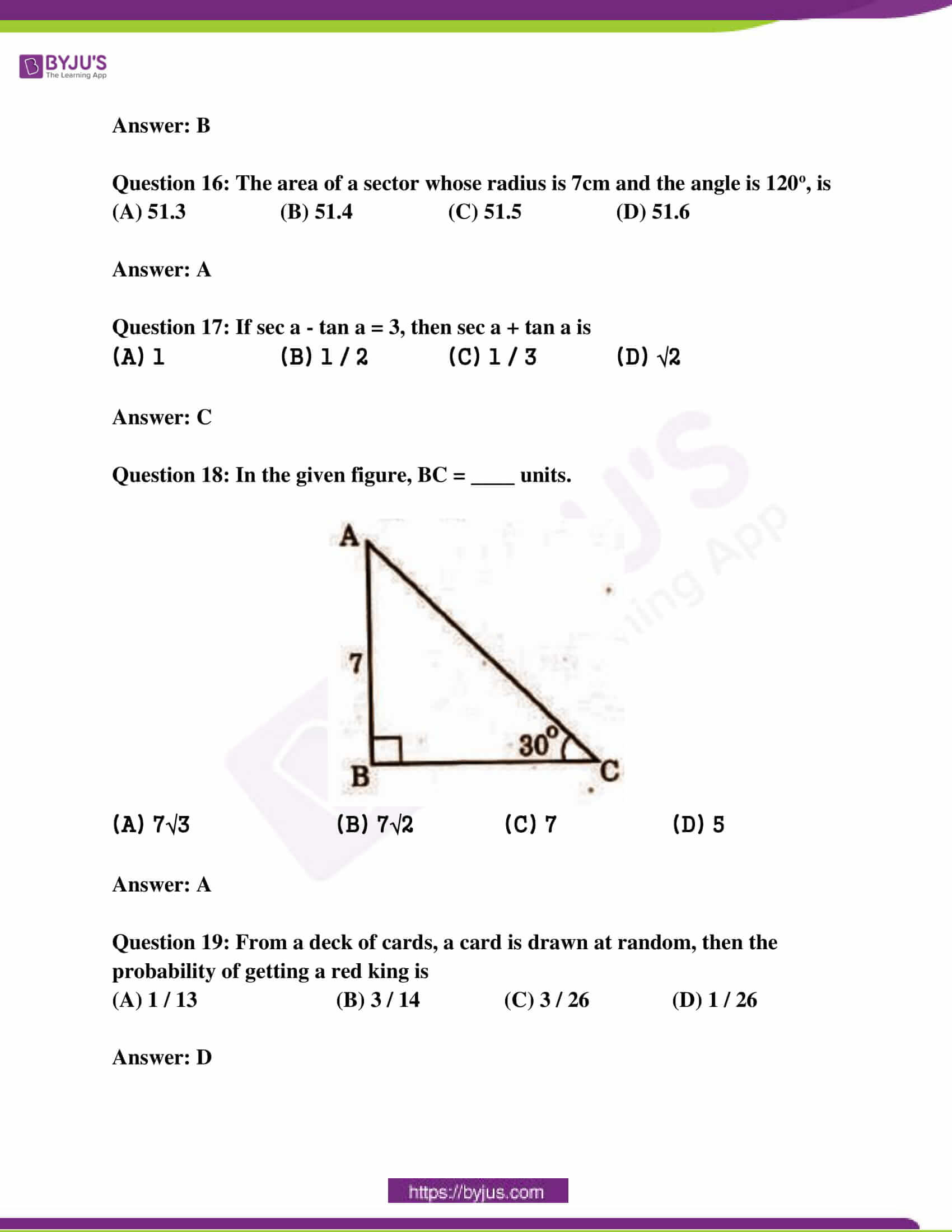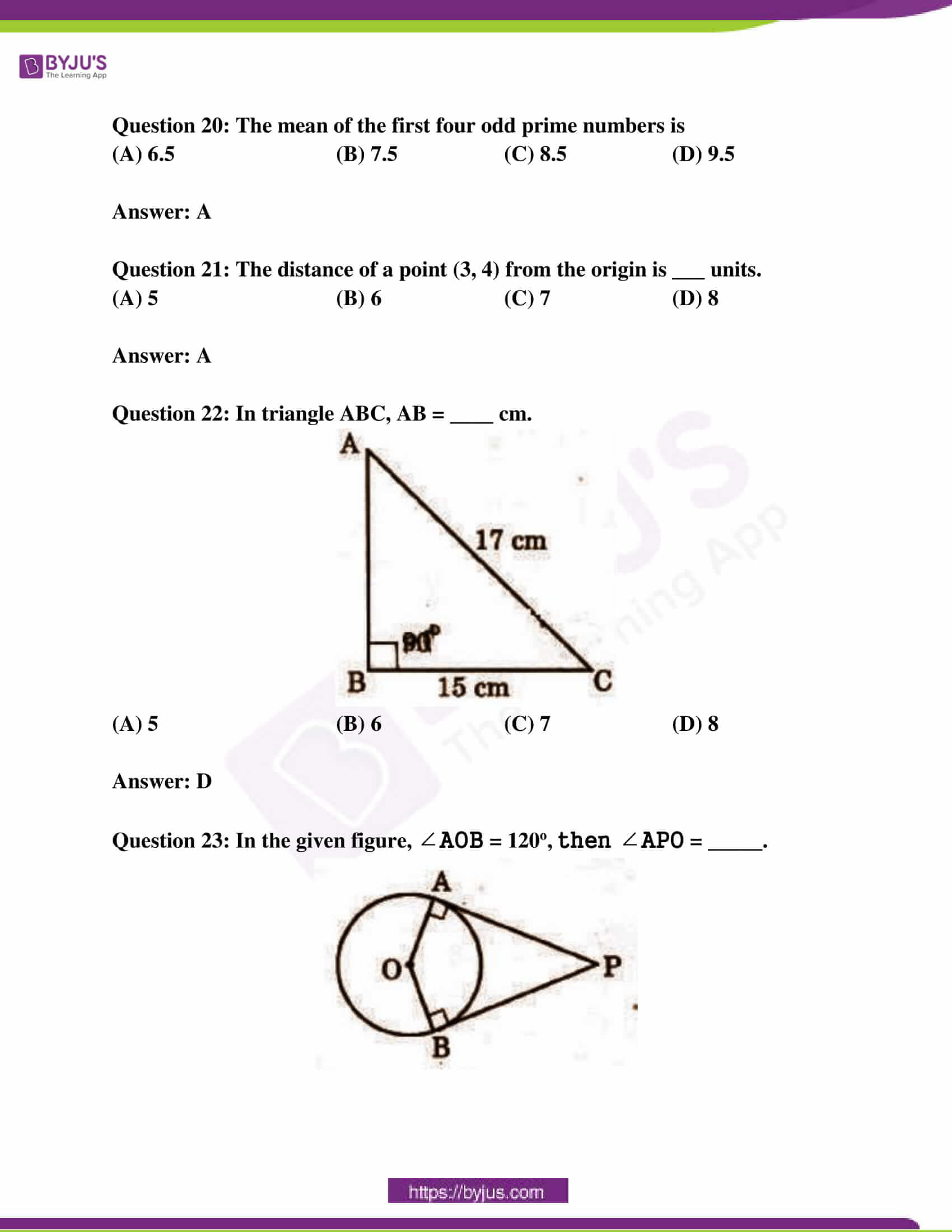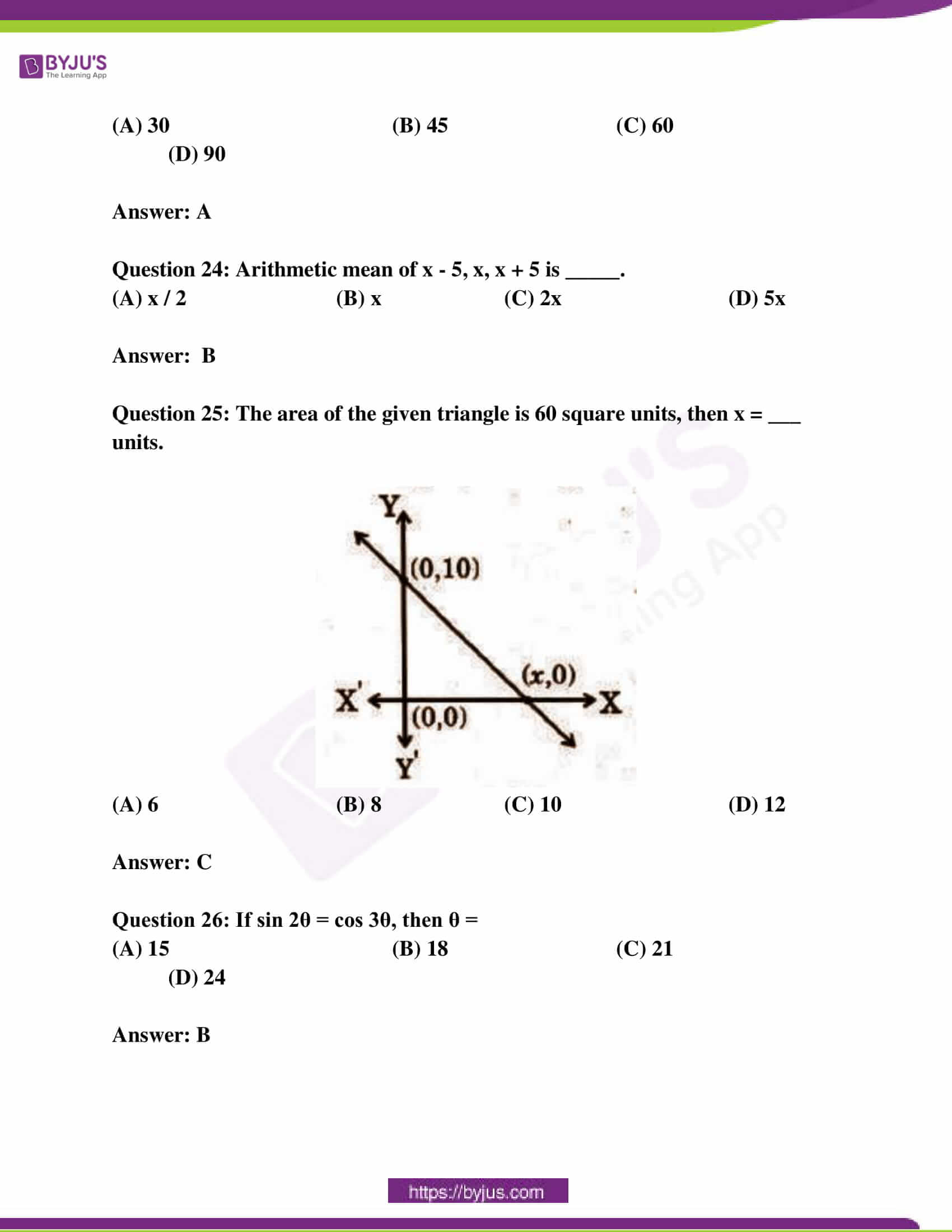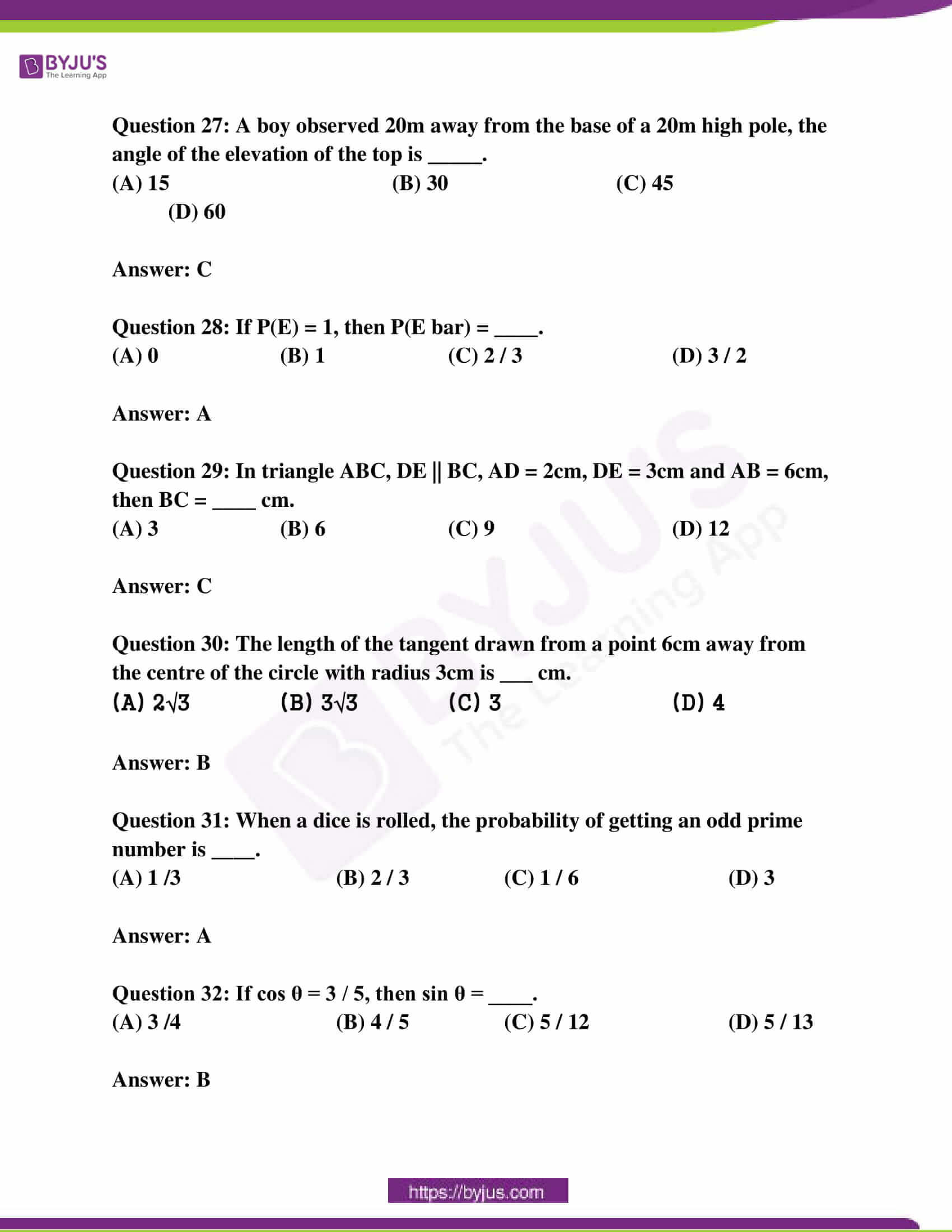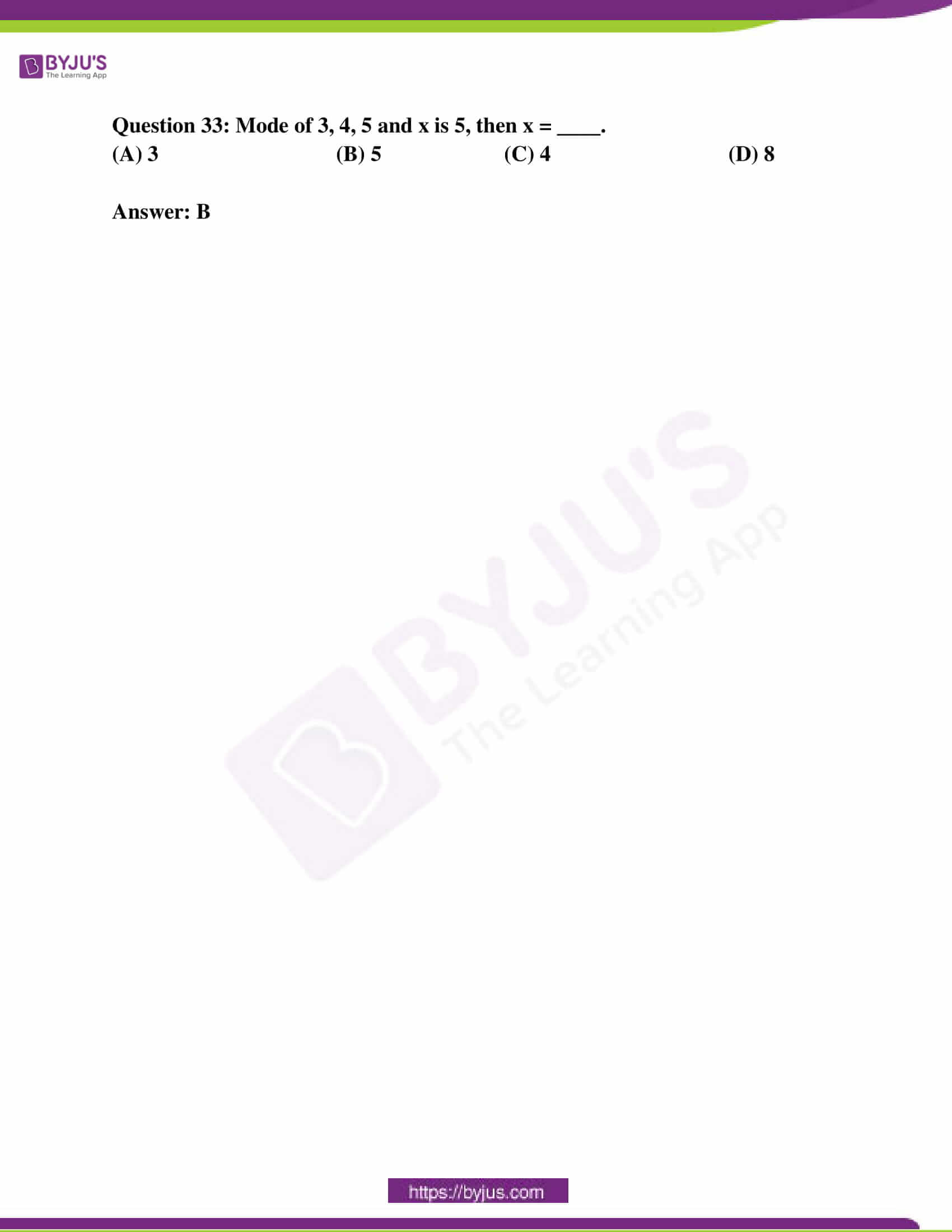SECTION – I

(4 * 1 = 4)

Question 1: If A (4, 0), B (o, y) and AB = 5, find the possible values of y.

Solution:

Given :- A (4,0) and B (0,y), and AB = 5

To find:- y

Distance formula = AB = √(x2 – x1)² + (y2 – y1

=> 5 = √(0 – 4)² + (y – 0)²

=> 5 = √(-4)² + (y²)

=> 5 = √16 + y²

Squaring on both sides

(5)² = (√16 + y²)²

=> 25 = 16 + y²

=> y² = 25 – 16

=> y² = 9

=> y = √9

=> y = ± 3

The possible values of y are 3 and -3.

Question 2: A boy observed from the top of an electric pole at an angle of elevation of 30o when the observation point is 10m away from the foot of the pole. Draw a suitable diagram for the above situation.

Solution:

Let the height of the electric pole AB be H.

Angle of elevation = 30o

The distance of observer form the base of pole BC = 10 m

Base = 10

Perpendicular = H

tan θ = perpendicular / base

tan 30o = H / 10

1 / √3 = H / 10

10 / √3 = H

So, Height of pole is 10 / √3 m

The figure is as follows: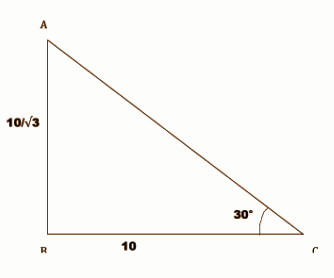Question 3: Find the value of tan2 45o + cot2 30o.

Solution:

tan2 45 + cot2 30

From the trigonometric values,

tan 45 = 1 and cot 30 = √3

Substitute,

tan2 45 + cot230

= (tan 30)2 + (cot 45)2

= (1)2 + (√3)2

= 1 + 3

= 4

Question 4: If P(E) = 0.546, what is the probability of P(not E)?

Solution:

P(E) = 0.546

P(not E) = 1 – 0.546

= 0.454

P(E’) = 0.454

SECTION – II

(5 * 2 = 10)

Question 5: Find the centroid of the triangle whose vertices are (-4, 4), (-2, 2) and (6, -6).

Solution:

Point 1 (x1, y1) = (-4, 4)

Point 2 (x2, y2) = (-2, 2)

Point 3 (x3, y3) = (6, -6)

Centroid of a Triangle =(x1 + x2 + x3 / 3, y1 + y2 + y3 / 3)

= (-4 – 2 + 6 / 3 , 4 + 2 – 6 / 3)

= (0 / 3 , 0 / 3)

= (0, 0)

Question 6: Triangle ABC ~ triangle DEF and their areas 64 and 121 cm2 respectively. If EF = 15.4cm, then find BC.

Solution:

Given that Triangle ABC ~ triangle DEF

The ratio of areas of two similar triangles is equal to the ratio of squares of any two corresponding sides.

Area of triangle ABC / Area of triangle DEF = BC2 / EF2

64 / 121 = BC2 / (15.4)2

64 / 121 = BC2 / 237.16

BC2 = 125.44

BC = 11.2 cm

Question 7: Prove that tan2 θ – sin2 θ = tan2 θ * sin2 θ.

Solution:

LHS = tan² θ – sin²θ

= sin²θ / cos²θ – sin²θ

= sin²θ [1 / cos²θ – 1] [we know, secx = 1 / cosx, so, 1 / cos²θ = sec²θ]

= sin²θ [ sec²θ – 1]

we also know, sec²x – tan²x = 1

so, sec²θ – tan²θ = 1

or, sec²θ – 1 = tan² θ

then, sin²θ [sec²θ – 1] = sin²θ × tan²θ = RHS

So, (tan²θ – sin²θ) = tan²θ × sin²θ

Question 8: A die is thrown once. Find the probability of getting

[a] an even number

[b] an odd prime number

Solution:

[a] The sample space has 6 possible outcomes – S = {1, 2, 3, 4, 5, 6}.

Probability of any one outcome occurring = 1/6

The event that an even number on tossing the die can occur in 3 possible ways.

Let E denote this event.

E = {2, 4, 6}

P(E) = 3 × (1 / 6) = 1 / 2

[b] The probable odd prime numbers appearing on a die roll are 3 and 5 (1 is not considered to be prime).

Since there are 6 possible outcomes, the probability of an odd prime is 2 out of 6

= 2 / 6

= 1 / 3

Question 9: Write less than cumulative frequency and greater than cumulative frequency table for the following data.

 CI 5 – 10 10 – 15 15 – 20 20 – 25 25 – 30 f 4 45 20 13 9

Solution:

 CI 5 – 10 10 – 15 15 – 20 20 – 25 25 – 30 f 4 45 20 13 9 Less than CF 4 49 69 82 91 Greater than CF 91 87 42 22 9

SECTION – III

(4 * 4 = 16)

Question 10:

[a] If cosec A + cot A = p, show that [p2 + 1] / [p2 – 1] = sec A.

OR

[b] Show that the points (-4, -7), (-1, 2), (8, 5) and (5, -4) taken in order are the vertices of a rhombus.

Solution:

[a]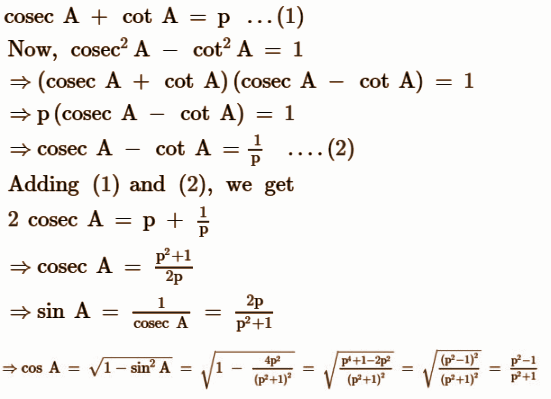[b] A (- 4, – 7), B (- 1, 2), C (8, 5), D (5, – 4)

XY = √(x2 – x1)² + (y2 – y1)² )

AB = √((-1 – (-4))² + (2 – (-7))²

= √(3² + 9²)

= √(90)

AB = 3√10

BC = √(8 – (-1))² + (5 – 2)²

= √9² + 3²

BC = 3√10

CD= √(5 – 8)² + (-4 – 5)²

CD= 3√10

AD= √(5 – (-4))² + (-4 – (-7))²

∴ AB = BC = CD = AD

AC = √(8 – (-4))² + (5 – (-7))²

= √144 + 144

AC = 12√2

BD = √(5 – (-1))² + (-4 – 2)²

= √36 + 36

BD = 6√2

∴AC ≠ BD

Since all the sides are equal and diagonals are not equal, it shows that the following vertices are of a rhombus.

Question 11:

[a] Find the mode of the following data.

 CI 50 – 52 53 – 55 56 – 58 59 – 61 62 – 64 f 15 110 135 115 25

OR

[b] A chord of a circle of radius 14cm subtends 120o angle at the centre. Find the area of the corresponding major segment of the circle.

Solution:

[a] The class interval is converted as follows

49.5 – 52.5

52.5 – 55.5

55.5 – 58.5

58.5 – 61.5

61.5 – 64.5

Maximum frequency = 135

Model class = 55.5 – 58.5

l = 55.5

f1 = 135

f2 = 115

f0 = 110

h = 3

mode = l + (f1 – f0 / 2f1 – f0 -f2) × h

= 55.5 + (135 – 110 / 2 × 135 – 110 – 115) × 3

= 55.5 + (25 / 270 – 225) × 3

= 55.5 + (25 / 45) × 3

= 55.5 + 25 / 15

= 55.5 + 5 / 3

= 55.5 + 1.67

Mode = 57.17

[b] Area of segment = (1 / 2) * (𝛉 – sin 𝛉) * r2, where 𝛉 = central angle, r = radius

𝛉 = 120o

In radian, 𝛉 = (120 / 180) 𝛑 = 2𝛑 / 3

r = 14 cm

Then, the area of the corresponding major segment of the circle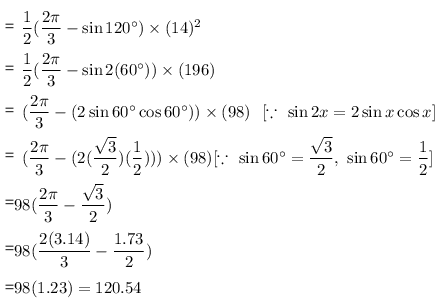The area of the corresponding major segment of the circle is 120.54 sq. cm.

Question 12:

[a] A bag contains 20 discs, which are numbered from 1 to 20. If one disc is drawn at random from the bag, find the probability that it bears:

[i] an even number

[ii] prime number

[iii] multiple of 5

[iv] 2 digits odd number

OR

[b] The angle of elevation of the top of the building from the foot of the tower is 30o and the angle of elevation of the top of the tower from the foot of the building is 60o. If the tower is 30m high, find the height of the building.

Solution:

[a] All possible outcomes are: 1, 2, 3, 4, ….. , 20

Total number of discs = 20

∴ Total number of all possible outcomes = 20

(i) Favourable outcomes are: 2, 4, 6, 8,….., 20

Number of favourable outcomes = 10

∴ P(getting an even number) = 10 / 20 = 1 / 2

(ii) Favourable outcomes are: 2, 3, 5, 7, 11, 13, 17 and 19

Number of favourable outcomes = 8

∴ P(getting a prime number) = 8 / 20 = 2 / 5

(iii) Favourable outcomes are: 5, 10, 15 and 20

Number of favourable outcomes = 4

∴ P(getting a multiple of 5) = 4 / 20 = 1 / 5

(iv) Favourable outcomes are 11, 13, 15, 17 and 19.

Number of favourable outcomes = 5

∴ P(getting a two-digit odd number) = 5 / 20 = 1 / 4

[b] Height of building = AB

Height of tower = CD = 50 m

The angle of elevation of the top of a building from the foot of the tower is 30° i.e. ∠ACB =30°

The angle of elevation of the top of the tower from the foot of the building is 60° i.e. ∠DBC =60°

In ΔDBC

tan 𝛉 = perpendicular / base

tan 𝛉 = DC / BC

tan 60o = 30 / BC

√3 = 30 / BC

BC = 30 / √3

In triangle ABC,

tan 𝛉 = perpendicular / base

tan 𝛉 = AB / BC

tan 30o = AB / [30 / √3]

1 / √3 = √3AB / 30

AB = 30 / 3

AB = 10m

Thus the height of the building is 10 m.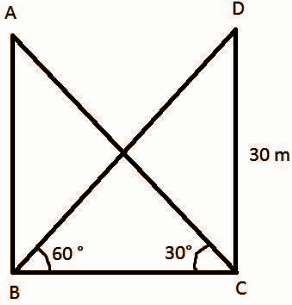Question 13:

[a] Construct a triangle similar to the given triangle ABC, with its sides equal to 3 / 4 of the corresponding sides of triangle ABC.

OR

[b] Draw a circle of radius 4cm. From a point 7.5cm away from the centre, construct a pair of tangents to the circle.

Solution:

[a]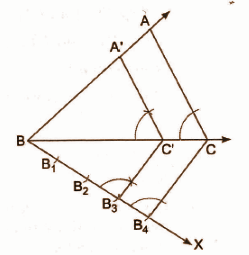[b]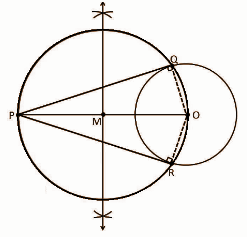SECTION – IV

(20 * 0.5 = 10)

Question 14: Slope of the line passing through the points (0, sin 60o) and (cos 30o, 0) is

(A) 0 (B) 1 (C) -1 (D) √3

Question 15: △ABC ~ △PQR and ∠A + ∠B = 115o, then ∠R =

(A) 55 (B) 65 (C) 75 (D) 45

Question 16: The area of a sector whose radius is 7cm and the angle is 120o, is

(A) 51.3 (B) 51.4 (C) 51.5 (D) 51.6

Question 17: If sec a – tan a = 3, then sec a + tan a is

(A) 1 (B) 1 / 2 (C) 1 / 3 (D) √2

Question 18: In the given figure, BC = ____ units.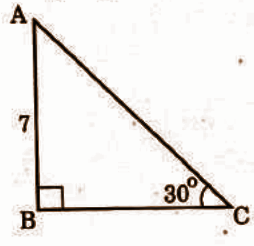(A) 7√3 (B) 7√2 (C) 7 (D) 5

Question 19: From a deck of cards, a card is drawn at random, then the probability of getting a red king is

(A) 1 / 13 (B) 3 / 14 (C) 3 / 26 (D) 1 / 26

Question 20: The mean of the first four odd prime numbers is

(A) 6.5 (B) 7.5 (C) 8.5 (D) 9.5

Question 21: The distance of a point (3, 4) from the origin is ___ units.

(A) 5 (B) 6 (C) 7 (D) 8

Question 22: In triangle ABC, AB = ____ cm.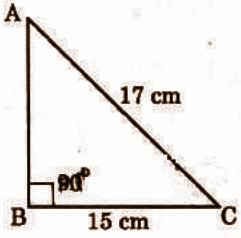(A) 5 (B) 6 (C) 7 (D) 8

Question 23: In the given figure, ∠AOB = 120o, then ∠APO = _____.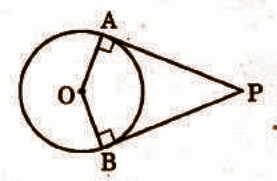(A) 30 (B) 45 (C) 60 (D) 90

Question 24: Arithmetic mean of x – 5, x, x + 5 is _____.

(A) x / 2 (B) x (C) 2x (D) 5x

Question 25: The area of the given triangle is 60 square units, then x = ___ units.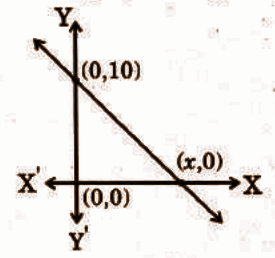(A) 6 (B) 8 (C) 10 (D) 12

Question 26: If sin 2θ = cos 3θ, then θ =

(A) 15 (B) 18 (C) 21 (D) 24

Question 27: A boy observed 20m away from the base of a 20m high pole, the angle of the elevation of the top is _____.

(A) 15 (B) 30 (C) 45 (D) 60

Question 28: If P(E) = 1, then P(E bar) = ____.

(A) 0 (B) 1 (C) 2 / 3 (D) 3 / 2

Question 29: In triangle ABC, DE || BC, AD = 2cm, DE = 3cm and AB = 6cm, then BC = ____ cm.

(A) 3 (B) 6 (C) 9 (D) 12

Question 30: The length of the tangent drawn from a point 6cm away from the centre of the circle with radius 3cm is ___ cm.

(A) 2√3 (B) 3√3 (C) 3 (D) 4

Question 31: When a dice is rolled, the probability of getting an odd prime number is ____.

(A) 1 /3 (B) 2 / 3 (C) 1 / 6 (D) 3

Question 32: If cos θ = 3 / 5, then sin θ = ____.

(A) 3 /4 (B) 4 / 5 (C) 5 / 12 (D) 5 / 13